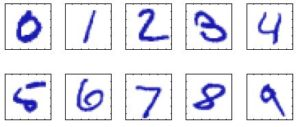# Machine Learning 101 – Overview of Machine Learning and Some Basic Terms

The is lesson 2 of our Machine Learning 101 course. This follows from Lesson 1.

We would cover the following:

To get started, I would like to state the basic goal of machine learning.

#### 1. The Goal of Machine Learning

The goal of machine learning is to create models that could perform actions that is generally performed by humans.

For example:

• understand and respond to spoken instructions
• understand handwritten text
• recognize possible danger (e.g fire)
• drive a car
• recognize a someone’s face in a photo
• predict the population of a country in some future year
• predict the stock market trend
• play a game with someone
• and so on

#### 2. How Machine Learning Works

To understand how machine learning works, first think of how the compute works.

As shown in Figure 1, a computer system takes some input (or inputs), passes it through the processor and produces an output. So there are three components:

• input
• processor (the computer)
• output

Now, in the case of machine learning, we provide an input and the output, then the processor(model) is the end result.

This is illustrated in Figure 2.

This is interesting because, it seems that the computer can build itself simply by looking at some existing inputs and outputs!

This also implies that  this model could modify itself. So if the input changes, it could rewrite it’s code to understand it.

#### 3. Handwritten Digits Example

Let’s say we want a system to understand handwriting. Handwritten digits of 1 to 10, for example. This is shown below. For humans, it is very trivial but for the computer, this is tough nut.

Each of the digit is in a square of that has 28 x 28 pixel image. Therefore, each image can be represented as 780 numbers (that is 28 x 28). In Machine learning terms, we can say each images is represented by a vector x of 784 numbers.

So in this case we want a model that would take this vector and tell us what number it is ( from 0 to 9).How to we proceed? Based on our example, we need to provide the input and output. But let’s look at the Machine Learning approach. Then you will also understand some useful Machine Learning terms. At least, for a start.

• The input in this case would be:  set of vectors (each representing a number)
• The output would be: a model that takes these vectors and gives us  the actual number
• The output would be the actual number that matches each input

Let’s clarify this as we discuss the Machine Learning terms you need to know

#### 4. Machine Learning Terms

To get our task done, we need  a large set of N handwritten digits {x1, x2,…, xn}. This is called the training set. For each of the handwritten digit(768 vector), we provide the actual number it corresponds to. This means that we also need a set of target vectors. These represents that actual identity of each digit.

So note the two terms you’ve learnt:

• Training Set
• Target Vector

To Illustrate: Assuming you want search dog to find a escaped fugitive. The search dog can do this by following his scent. So the the first thing you do is the provide say, the cloth of this fugitive to the dog. Once  the dog ‘learns’ the fugitive’s scent, then he can track him.

In the same way we provide a training set and a corresponding test set. We use this data to train the model (this process is also called learning or training). That is where the ‘Learning’ in machine learning comes from.

Once the model is trained, we need to test it. We do this by providing a few handwritten digits and allowing the model to predict the actual digit. This few test data is called the Test Data Set.

Now you have learnt two more terms:

• Learning (training)
• Test Data set (or test set)

After testing, you can now use the model. The ability of your model to categorize correctly is called generalization.

Remember that the initial digits are placed inside a 28 x 28 pixels box. This is a kind of preprocessing (or transformation) to make the task a bit easier to solve.

The idea of transforming the input data is also known a feature extraction. This helps to reduce the variability within each digit.

Now you have learnt two more terms:

• preprocessing
• feature extraction

At this point, I would allow you to digest what we’ve covered so far. In the next class, we would look at classes of Machine Learning problems.#### kindsonthegenius

Kindson Munonye is currently completing his doctoral program in Software Engineering in Budapest University of Technology and Economics

View all posts by kindsonthegenius →